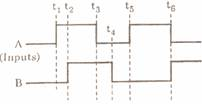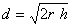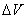Wednesday 25th November 2020

CBSE Guess > Papers > Question Papers > Class XII > 2005 > Physics > Compartment Delhi Set-II

PHYSICS 2005 (Set II—Compartment Delhi)

Except fort the following questions, all the remaining questions have been asked in Set I. .

Q. 1. What is a transponder? 1

Q. 2. Name one impurity each, which when added to pure Si, produces (i) n-type and (ii) p-type semiconductor. 1

Q. 3. Name the physical quantity whose S I unit is Weber. Is it a scalar or a vectoer quantity? 1

Q. 6. Draw a labelled diagram of Lechlanche cell. What is the function of ammoni- um chloride used in it? 2

Q. 7. For two given input wave forms A and B,sketch the output wave from obtained from AND gate. Draw its logic symbol. 2

Q. 8. Show the biasing of a photo-diode with the help of a circuit diagram. Draw graphs to show variations in reverse bias currents for different illumination intensities. 2

Q. 10. Using the data given below, state as to which of the given lenses will you prefer to use as (i) an eye-piece, and (ii) an objective, to design a compound microscope. Give reason for your answer. 2

 lens Power Aperture A 20 D 0.02 m B 10 D 0.02 m C 10 D 0.05 m D 1.0 D 0.1 m

Q. 13. Show that the range of transmission 'd' of a T.V. tower of height 'h' is given by the relation, where r is the radius of the earth.

A ground receiver station is receiving a signal at (a) 5 MHz, and (b) 100 MHz, transmitted from a ground transmitter at a height of 300 km located at a dista- nce of 100 km. Identify whether it is coming via space wave, sky wave propagation or satellite transponder. Radius of the earth = 6.4 x 106 m; the maximum electron density per m 3 (Nmax) of ionosphere = 1012 . 3

Q. 14. With the help of a labelled diagram, describe briefly the construction of a coaxial cable. Name two factors on which the power transmitted through a coaxial cable depends. What is the upper limit of frequency upto which a coaxial cable can be used? 3

Q. 20. A short bar magnet placed with its axis inclined at 300 to the external magnetic field of 800 G acting horizontally experiences a torque of 0.016 Nm. Calculate (i) the magnetic moment of the magnet, (ii) the work done by an external force in moving it from most stable to most unstable position. (iii) What is the work done by the force due to the external magnetic field in the process mentioned in (ii)? 3

Q. 24. A parallel plate capacitor of plate separation 'd' is charged to a potential difference. dielectric slab of thickness 'd' and dielectric constant 'K' is introduced between the plates while the battery remains connected to the plates.

(i) Find the ratio of energy stored in the capacitor after and before the dielec- tric is introduced. Give the physical explanation for this change in stored energy.

(ii) What happens to the charge on the capacitor? 3

Physics 2005 Question Papers Class XII

CBSE 2005 Question Papers Class XII